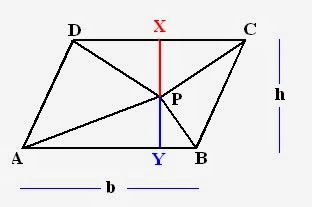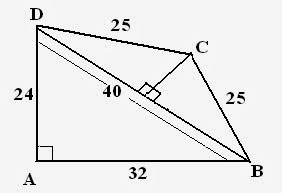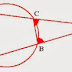In my previous session, I have discussed some concepts related to triangles. Today I will discuss some important questions of Geometry which used to appear in SSC exams. Generally, questions asked from this section are based on properties of various shapes like lines, angles, triangles, rhombus, circles etc.

## Important Examples

Example1: ABCD  is a cyclic quadrilateral. AB and CD are produced to meet P. If angleADC = 70^o and angle DAB = 60^o, then what will be angle PBC + angle PCB?

Solution: First step: Make an appropriate figure using statements provided in  questions.• Cyclic quadrilateral has all its vertices on circle and sum of all angles is 360^o.
• Sum of opposite angles = 180^o.
• External angle = Opposite internal angle.
Therefore, angle PBC = 70^o
angle PCB = 60^o

So, PBC + PCB = 130^0

See its very simple question, if you know the properties of geometry.Lets try more examples

Example2: ABCD is a parallelogram and P is any point within it. If area of parallelogram is 20 units, then what will be the sum of areas of triangle PAB and PCD?

Solution: According to the question, figure will be as follows:Properties for this question:
• Area of parallelogram = Base times Height.
• Area of triangle = 1/2 Base times altitude.
Given that: Area of parallelogram = 20
i.e. Base times Height = 20
⇒ b times h = 20

To find =  Area of ( PAB +PCD)
⇒ (1/2) b times (PY) + (1/2) b times (PX)                          ( See Figure)

⇒1/2 b ( PX +PY)

⇒1/2 b h
⇒(1/2) times 20
⇒10 units.

Example3: ABCD is a cyclic trapezium with AB parallel DC and AB diameter of circle. If angle CAB = 30^o, then angle ADC will be?

Solution: According to ques, figure will be as follows:• Angle subtended by diameter is always 90^o.
• Sum of angles of triangle = 180^o.
• Sum of opposite angles = 180^o
angleACB = 90^o
angle BAC = 30^o

Therefore,

angle ABC = 60^o

angle ABC + angle ADC = 180^o
⇒ 60^o + ADC = 180^o
⇒ angle ADC = 120^o

Example4: Two side of plot measures 30m and 22m and angle between them is 90^o. The other two sides measures 24m and the three remaining angles are not right angles. Find the area of plot.

Solution: Figure becomes:
Center point of BD = OABD is a right angle triangle, therefore, Pythagoras theorem followed.

(BD)^2 = (AB)^2 + (AD)^2
⇒ BD = 40

Given that, BC = CD
Therefore, line drawn from C to BD given right angles
Also, this will lead to DO = BO

So, DO = BO = 20

Similarly, using Pythagoras theorem, OC = 15 m

Now, Area of triangles (ABD + BOC + COD)
⇒ 1/2 (24 times 32) + 2 ((1/2) 20 times 15)
⇒ 684 m^2

Important questions of Geometry for SSC CGL Tier IReviewed by Jasleen Behl on Saturday, February 15, 2014 Rating: 5
1.